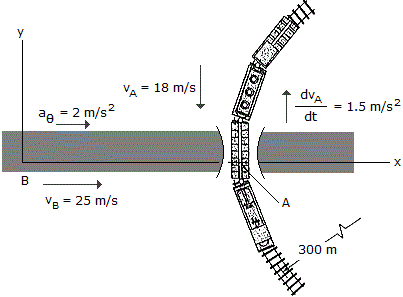# Engineering Mechanics - Kinematics of Particle (KOP) - Discussion

### Discussion :: Kinematics of Particle (KOP) - General Questions (Q.No.6)

6.A passenger in the automobile B observes the motion of the train car . At the instant shown, the train has a speed of 18 m/s and is reducing its speed at a rate of 1.5 m/s2. The automobile is accelerating at 2 m/s2 and has a speed of 25 m/s. Determine the velocity and acceleration of A with respect to B. The train is moving along a curve of radius = 300 m.

 [A]. vA/B = (25.0i+18.00j) m/s, aA/B = (2.00i-1.500j) m/s2 [B]. vA/B = (25.0i+18.00j) m/s, aA/B = (0.920i-1.500j) m/s2 [C]. vA/B = (-25.0i-18.00j) m/s, aA/B = (-2.00i+1.500j) m/s2 [D]. vA/B = (-25.0i-18.00j) m/s, aA/B = (-0.920i+1.500j) m/s2

Explanation:

No answer description available for this question.

 Rohit Kumar said: (Apr 19, 2015) Train is moving in circle so it has 2 accelerations. One along tangent and one along center. So in calculating relative acceleration we have to consider both acceleration of train in x and y direction which is centripetal and tangential acceleration simultaneously.

 Abel said: (Apr 21, 2016) How to solve this problem? Please explain the steps.

 Johnn said: (Oct 20, 2018) How to solve this problem? Please explain the steps.

 Nagesh said: (Apr 4, 2022) Please explain the step of the calculation.